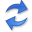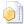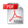Telling Cause from Effect using Local and Global Regression

Abstract. We consider the fundamental problem of inferring the causal direction between two univariate numeric random variables $$X$$ and $$Y$$ from observational data. The two-variable case is specifically interesting as because the graph $$X$$ causes $$Y$$, and vice versa, are Markov equivalent, and hence it is not possible to determine the correct direction using standard approaches that rely on conditional independence tests.

To tackle this problem, we follow an information theoretic approach based on the algorithmic Markov condition and use the Minimum Description Length (MDL) principle to provide a practical solution. In a nutshell, in this paper we perform causal inference by compression. We infer that $$X$$ is a likely cause of $$Y$$ when we find that we find we need fewer bits to describe the data over $$X$$ and $$Y$$ more succinctly by first transmitting the data over $$X$$, and then the data of $$Y$$ as a function of $$X$$, than for the inverse direction.

To put this notion to practice, we propose an MDL score to determine how many bits we need to transmit the data using a class of regression functions that can model both local and global functional relations. To determine whether an inference, i.e. the difference in compressed sizes, is significant, we propose two analytical significance tests based on the no-hypercompression inequality. Last, but not least, we introduce Slope, a linear-time algorithm that through thorough empirical evaluation on both synthetic and real world data we show outperforms the state of the art by a wide margin.

Important Slope has been superceded by Sloppy

## Implementationthe R source code (December 2018) by Alexander Marx.

## Related PublicationsMarx, A Information-Theoretic Causal Discovery. Dissertation, Saarland University, 2021.Marx, A & Vreeken, J Telling Cause from Effect by Local and Global Regression. Knowledge and Information Systems vol.60(3), pp 1277-1305, IEEE, 2019. (IF 2.397)Marx, A & Vreeken, J Telling Cause from Effect by MDL-based Local and Global Regression. In: Proceedings of the IEEE International Conference on Data Mining (ICDM'17), pp 307-316, IEEE, 2017. (full paper, 9.3% acceptance rate; overall 19.9%)